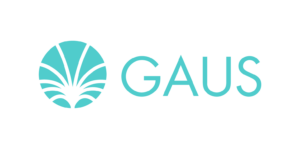# Geometry and Arithmetic of Uniformized Structures​

### Geometry and Arithmetic of Uniformized StructuresThe concept of uniformization is ubiquitous in mathematics. It serves as a tool to replace a complicated geometric object by a simpler one without altering the local structure. The original mathematical complexity is now encoded in a suitable symmetry group. This translation into another language opens up new perspectives for the study of the original mathematical objects. A very active and successful area of research uses this rich framework to study the geometry and arithmetic of algebraic varieties.

Important features in the concept of uniformized spaces include the following: automorphic forms arise as functions respecting these symmetry groups. Galois representations, which are fundamental in all branches of modern number theory, encode arithmetic symmetries. The tower of all covering spaces between the original object and its uniformization reflects the ‘paths’ of the space under consideration and ultimately leads to topological and cohomological invariants that are often of a non-abelian nature. Frobenius symmetry enhances geometry in the parallel universe of positive characteristic in an unexpected manner. Celebrated new results by Fields medallist Peter Scholze provide a bridge from the classical setting to the realm of positive characteristic, thus allowing the application of Frobenius symmetry even to classical questions in the framework of uniformization.

The core areas of the CRC/TRR are:

(A) Moduli spaces and automorphic forms
(B) Galois representations and étale invariants
(C) Cohomological structures and degeneration in positive characteristic